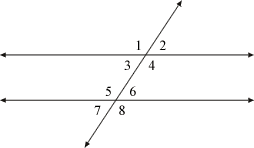index: click on a letter A B C D E F G H I J K L M N O P Q R S T U V W X Y Z A to Z index index: subject areas numbers & symbols sets, logic, proofs geometry algebra trigonometry advanced algebra & pre-calculus calculus advanced topics probability & statistics real world applications multimedia entrieswww.mathwords.com about mathwords website feedback

Alternate Exterior Angles

In the drawing below, angles 1 and 8 are alternate exterior angles, as are angles 2 and 7. Alternate exterior angles are congruent. Formally, alternate exterior angles are defined as two exterior angles on opposite sides of a transversal which lie on different parallel lines.

 Parallel lines cut by a transversal